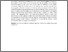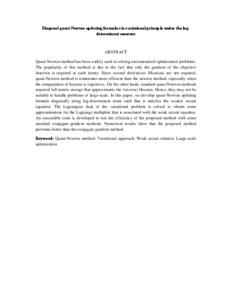# Diagonal quasi-Newton updating formula via variational principle under the log-determinant measure

## Citation

Sim, Hong Seng and Leong, Wah June and Chen, Chuei Yee and Ibrahim, Siti Nur Iqmal (2015) Diagonal quasi-Newton updating formula via variational principle under the log-determinant measure. In: International Conference on Control, Optimization and Autonomous Vehicles 2015 (COAV2015), 30-31 July 2015, Putrajaya, Malaysia. (p. 21).

## Abstract

Quasi-Newton method has been widely used in solving unconstrained optimization problems. The popularity of this method is due to the fact that only the gradient of the objective function is required at each iterate. Since second derivatives (Hessian) are not required, quasi-Newton method is sometimes more efficient than the newton method, especially when the computation of hessian is expensive. On the other hand, standard quasi-Newton methods required full matrix storage that approximates the (inverse) Hessian. Hence, they may not be suitable to handle problems of large-scale. In this paper, we develop quasi-Newton updating formula diagonally using log-determinant norm such that it satisfies the weaker secant equation. The Lagrangian dual of the variational problem is solved to obtain some approximations for the Lagrange multiplier that is associated with the weak secant equation. An executable code is developed to test the efficiency of the proposed method with some standard conjugate-gradient methods. Numerical results show that the proposed method performs better than the conjugate gradient method.Preview
Text (Abstract)
Diagonal quasi-Newton updating formula via variational principle under the log-determinant measure.pdfView Item